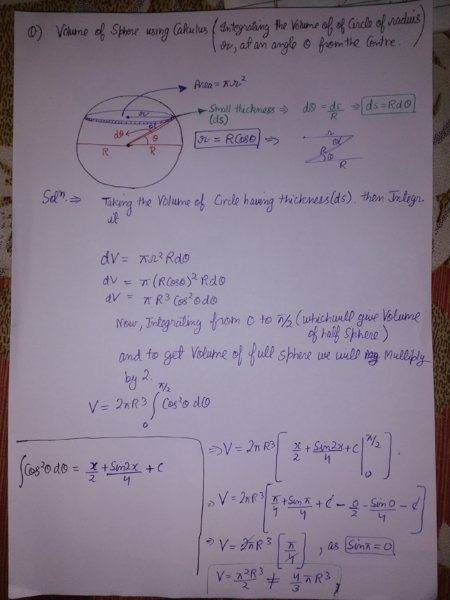# Derive the Volume of a Sphere using Calculus

• Shivam
Homework Statement Derive the volume of sphere using Calculus. i saw videos on this topic on youtube, but i want to do it by the method of integrating a circle at angle θ (Theta) . i am posting a photo where i explained every thing i did but i couldn't know what i am doing wrong.Homework EquationsIntegrating a circle of radius r at angle θ (Theta)The Attempt at a Solution https://drive.google.com/open?id=1Va32w9eCJE_2nH4VIPqG8bFHRzcKuvIF[/B]The key to understanding this is to look at a cross section of the sphere, which is a circle.

## Homework Statement

Derive the volume of sphere using Calculus. i saw videos on this topic on youtube, but i want to do it by the method of integrating a circle at angle θ (Theta) . i am posting a photo where i explained every thing i did but i couldn't know what i am doing wrong.

## Homework Equations

Integrating a circle of radius r at angle θ (Theta)

## The Attempt at a Solution#### Attachments

Shivam said:
i am posting a photo where i explained every thing i did but i couldn't know what i am doing wrong.

Also, you should make the effort of typing out your attempt if you want people to help you.

Your error is using ##ds## for the thickness of your disk. It should be ##dy=R\cos\theta d\theta##.

•Shivam
Shivam said:

## Homework Statement

Derive the volume of sphere using Calculus. i saw videos on this topic on youtube, but i want to do it by the method of integrating a circle at angle θ (Theta) . i am posting a photo where i explained every thing i did but i couldn't know what i am doing wrong.

## Homework Equations

Integrating a circle of radius r at angle θ (Theta)

## The Attempt at a Solution

I can see your attempted solution. The problem is with ##ds=Rd\theta##. That is arc length along the surface of the sphere. It is not the same as the thickness of your circular section. Can you correct it?

Shivam said:

## Homework Statement

Derive the volume of sphere using Calculus. i saw videos on this topic on youtube, but i want to do it by the method of integrating a circle at angle θ (Theta) . i am posting a photo where i explained every thing i did but i couldn't know what i am doing wrong.

## Homework Equations

Integrating a circle of radius r at angle θ (Theta)

## The Attempt at a Solution

LCKurtz said:
Your error is using ##ds## for the thickness of your disk. It should be ##dy=R\cos\theta d\theta##.
I got the correct answer by using the correcct thickness you gave me , but i still don't know how did you get that, can you explain please.

Shivam said:
I got the correct answer by using the correcct thickness you gave me , but i still don't know how did you get that, can you explain please.

The thickness is the vertical thickness of the slice. The arc length you have is not vertical, it's tangent to the sphere. So it makes a varying angle with the vertical as you move up the sphere. Use trig to turn that into a vertical distance.

•Shivam
LCKurtz said:
Your error is using ##ds## for the thickness of your disk. It should be ##dy=R\cos\theta d\theta##.

Can you show me how did you get that, i thought all day but i can't get it.

Shivam said:
Can you show me how did you get that, i thought all day but i can't get it.
Well, you have ##y=R \sin(\theta)##, so ##\frac{dy}{d\theta}=R \cos(\theta)##, so rearranging the differentials gives ##dy=R \cos(\theta)d\theta##. Also, try to understand this result from a geometrical point of view (i.e. how does a small increase in the angle influece the increase in y?)

•Shivam